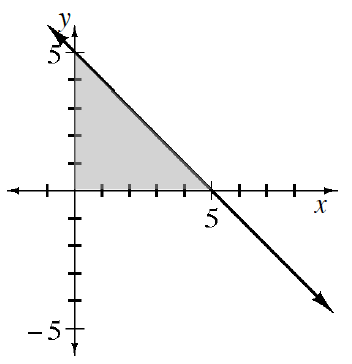### Home > APCALC > Chapter 1 > Lesson 1.1.1 > Problem1-4

1-4.

Examine the graph the function $f (x) = 5 - x$ at right.

Calculate the area of the shaded region.

Notice the familiar geometric shape.

Notice that the line dips below the $x$-axis when $x > 5$. When you are asked to calculate the “area under a curve” this refers to the region between the curve and the $x$-axis. Any area below the $x$-axis is considered to be negative. Calculate the area under the curve for $0 ≤ x ≤ 7$.

Find the area of the negative region and combine
it with the positive area found in part (a).

$A (f, 0 ≤ x ≤ 5) + A (f, 5 ≤ x ≤7)$

Determine the value of k such that the area under the curve for $0 ≤ x ≤ k$ is $0$. Show how you obtained your answer clearly and completely.

$A (f, 0 ≤ x ≤ 5) + A (f, 5 ≤ x ≤ k)$

$A(f,0\leq x\leq 5)+A(f,5\leq x\leq k)=$

$[\text{area from part a}]+\frac{1}{2}(k-5)(f(k))=$

$[\text{area from part a}]+\frac{1}{2}(k-5)(-k+5)=\underline{ \ \ \ \ \ \ \ \ \ \ \ \ \ \ \ \ \ \ \ \ \ \ \ \ \ \ \ \ }$